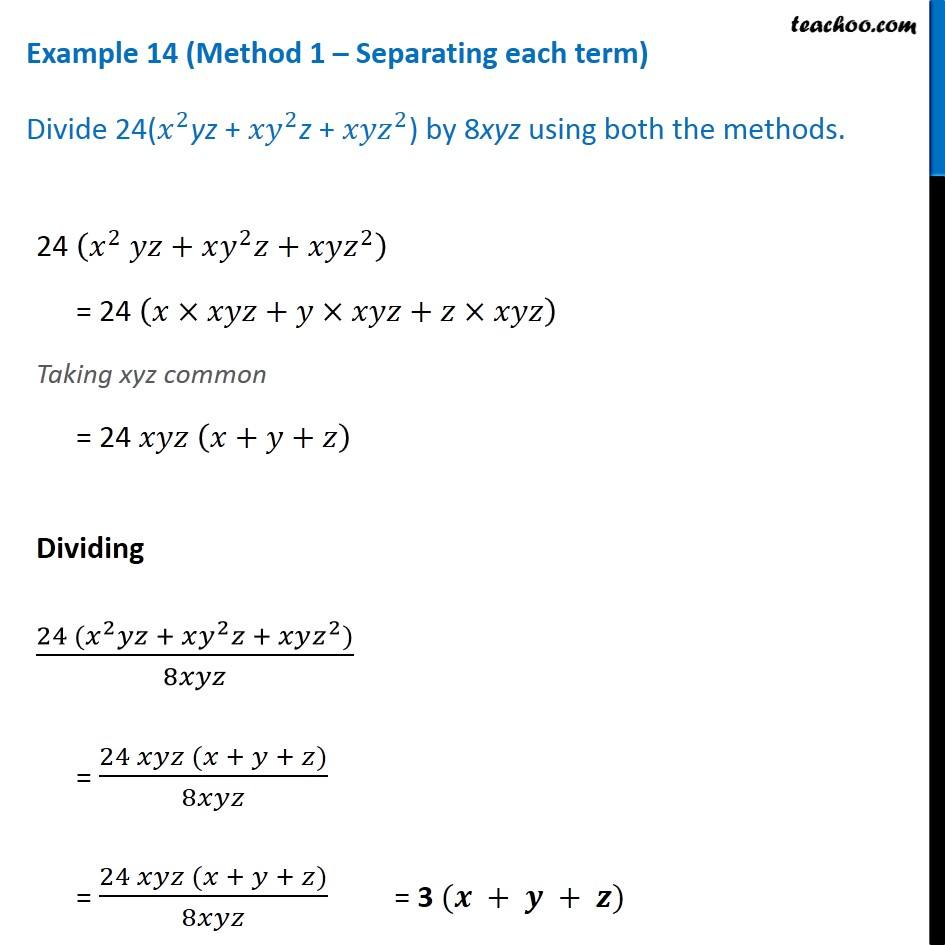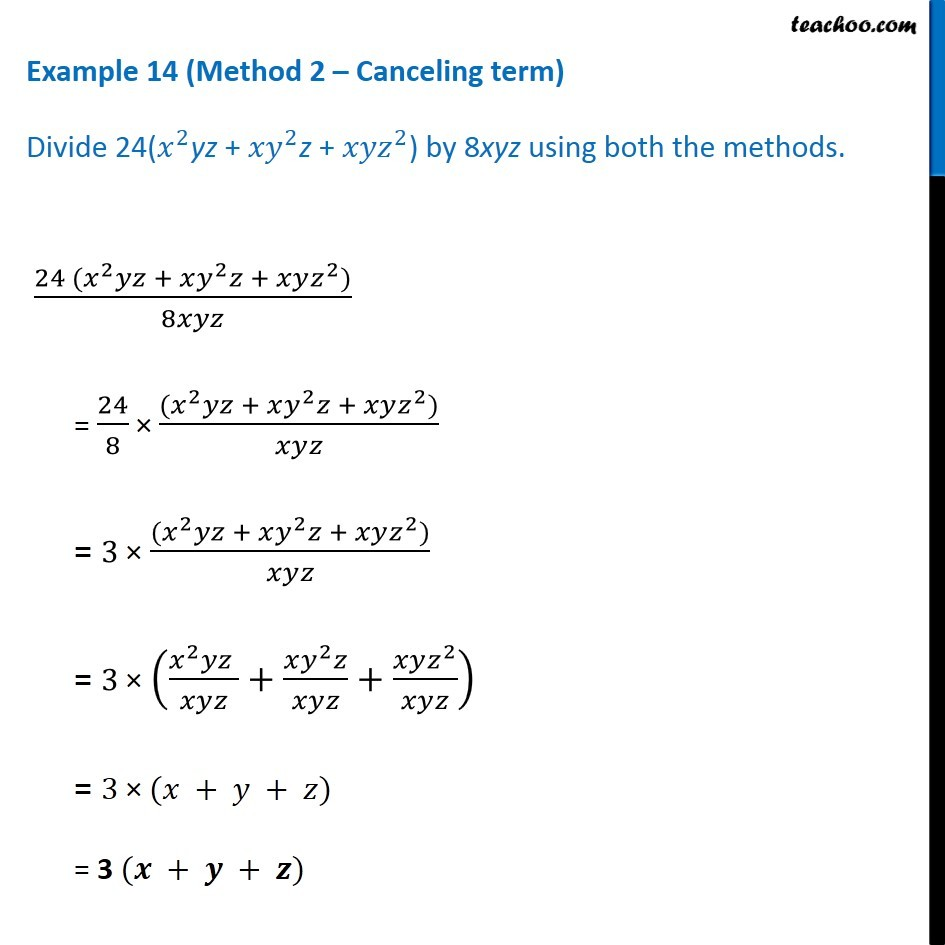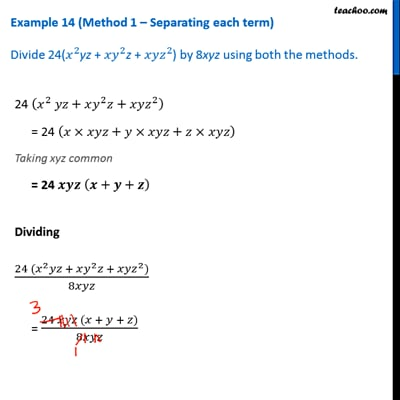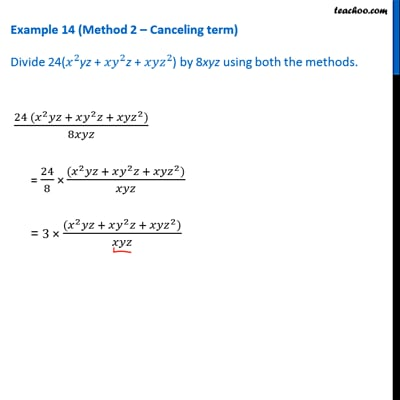Examples

Chapter 14 Class 8 Factorisation
Serial order wiseThis video is only available for Teachoo black usersThis video is only available for Teachoo black users

Get live Maths 1-on-1 Classs - Class 6 to 12

### Transcript

Example 14 (Method 1 – Separating each term) Divide 24(𝑥^2yz + 〖𝑥𝑦〗^2z + 〖𝑥𝑦𝑧〗^2) by 8xyz using both the methods. 24 (𝑥^2 𝑦𝑧+𝑥𝑦^2 𝑧+𝑥𝑦𝑧^2 ) = 24 (𝑥×𝑥𝑦𝑧+𝑦×𝑥𝑦𝑧+𝑧×𝑥𝑦𝑧) Taking xyz common = 24 𝑥𝑦𝑧 (𝑥+𝑦+𝑧) Dividing (24 (𝑥^2 𝑦𝑧 + 𝑥𝑦^2 𝑧 + 𝑥𝑦𝑧^2))/8𝑥𝑦𝑧 = (24 𝑥𝑦𝑧 (𝑥 + 𝑦 + 𝑧))/8𝑥𝑦𝑧 = (24 𝑥𝑦𝑧 (𝑥 + 𝑦 + 𝑧))/8𝑥𝑦𝑧 = 3 (𝒙 + 𝒚 + 𝒛) Example 14 (Method 2 – Canceling term) Divide 24(𝑥^2yz + 〖𝑥𝑦〗^2z + 〖𝑥𝑦𝑧〗^2) by 8xyz using both the methods. (24 (𝑥^2 𝑦𝑧 + 𝑥𝑦^2 𝑧 + 𝑥𝑦𝑧^2))/8𝑥𝑦𝑧 = 24/8 × ((𝑥^2 𝑦𝑧 + 𝑥𝑦^2 𝑧 + 𝑥𝑦𝑧^2))/𝑥𝑦𝑧 = 3 × ((𝑥^2 𝑦𝑧 + 𝑥𝑦^2 𝑧 + 𝑥𝑦𝑧^2))/𝑥𝑦𝑧 = 3 × ((𝑥^2 𝑦𝑧 )/𝑥𝑦𝑧+(𝑥𝑦^2 𝑧)/𝑥𝑦𝑧+(𝑥𝑦𝑧^2)/𝑥𝑦𝑧) = 3 × (𝑥 + 𝑦 + 𝑧) = 3 (𝒙 + 𝒚 + 𝒛)